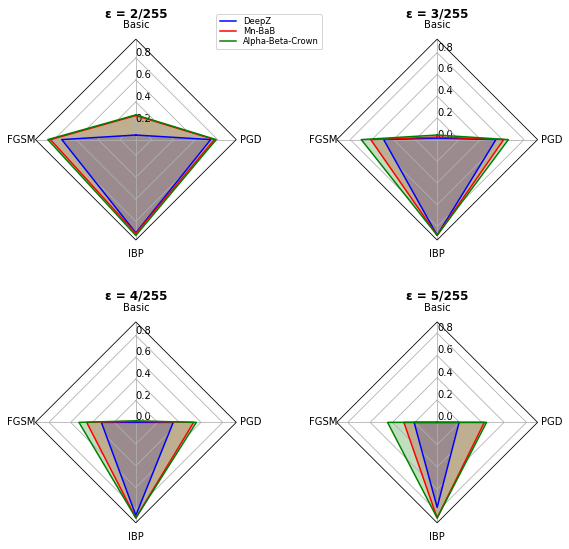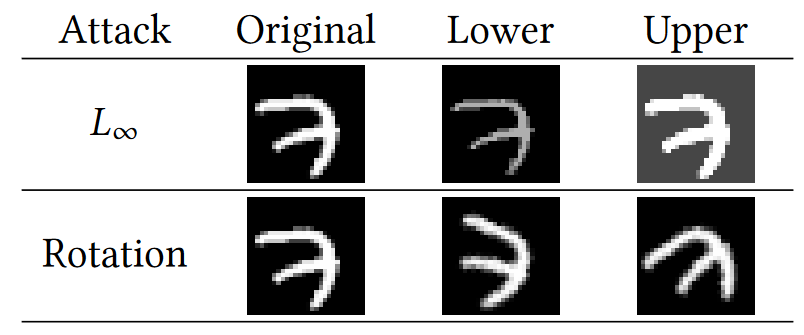Motivations

Artificial Intelligence (AI) is now present in many of the services we use, and is becoming increasingly so, particularly in mission-critical systems such as autonomous driving, aviation and medical imaging. At the same time, it has been discovered in recent years that these AI-based systems are sensitive to certain disturbances in their inputs or models. This weakness poses a problem precisely for mission-critical systems. Indeed, an autonomous car that fails to detect a pedestrian due to a disturbance is out of the question. So we need to test models using AI.

Testing techniques are widespread in the world of software engineering, but are not directly applicable to AI. In fact, in software that doesn’t use them, the programmer specifies the whole process, which follows a certain logic. On the other hand, in AI-based systems, the programmer describes the desired behavior of the system, but the logic is defined by the model trained on the data, as shown in the following figure.

To meet this demand for testing, we distinguish between two types of techniques: on the one hand, test methods that seek to highlight the system’s faults in relation to a set of cases (e.g., usage scenarios), and formal methods that use logic and mathematics to prove certain properties of the system and guarantee the absence of faults.

In this project, we studied formal verification for image classification neural networks.

How it works

Image classification involves taking an image as input and classifying it into one of a number of pre-defined categories. The neural networks most commonly used for image classification are convolutional networks, which combine convolution, pooling and fully-connected neural layers. At the output, the number of neurons corresponds to the number of possible classes, and the system returns the class with the highest probability, as in the following example:

The testing of such a system can be approached from the angle of robustness, using perturbed images as input. A first idea would be to manually modify the images and check whether the network predicts the same result. However, this would be far too time-consuming, as an infinite number of perturbation types and levels are possible. The advantage of formal verification is that it guarantees the robustness of a model for a range of disturbance levels. In practice, a perturbation corresponds to a decrease or increase in the pixel values of the image input to the network.

Methods

• Abstract interpretation

One formal verification method is abstract interpretation. This consists in bounding the values of the neurons by using abstract transformers to propagate the perturbation applied to the input of the network. This method allows us to obtain bounds around each class probability at the output, and to consider our AI robust for an image if the true class bound is separated from the others. This process is illustrated in the following diagram.

• Linear approximation

Another method is linear approximation. It closely resembles the abstract interpretation, but uses linear bounds around the neuron values instead of abstract transformers (see figure below).

In application, abstract interpretation and linear approximation have generally similar results.

Branch-and-Bound

Another method is Branch-and-Bound (BaB), which, as the name suggests, combines a bounding box approach with a sub-problem approach. A sub-domain is “verified” if its lower bound is greater than 0. The BaB procedure ends when all sub-domains have been verified, or when the allotted time has elapsed. If all subdomains are verified, the model is guaranteed to be robust to the perturbation described above for the image used. BaB can be used in conjunction with abstract interpretation and linear approximation to refine verification.

Case studies

Three variations of these methods are available:

• DeepZono, which uses abstract interpretation alone,
• Mn-BaB, which uses abstract interpretation coupled with BaB,
• and Alpha-Beta-CROWN, which uses linear approximation coupled with BaB.

These were used on the CIFAR10 dataset, which comprises some 60,000 images divided into 10 classes: airplane, car, bird, cat, deer, dog, frog, horse, boat, truck. The model used is a convolutional neural network (4 pooling-free convolution layers and 2 linear layers) for image classification trained in four ways:

• Classic;
• On antagonistic examples generated by FGSM
• On antagonistic examples generated by PGD
• Crown-IBP robustness training method
• Antagonistic examples are data perturbed with the aim of misleading an AI model. Many other methods for generating them exist in the literature.

ResultsRobustness level of models according to their training, observed on three applications under different levels of disturbance ε

The robustness level corresponds to the ratio of the number of images for which the model is certified robust to the number of images well classified by the model. Thus, a robustness level of 1 represents the case where the model is certified robust for all images well classified by it. A robustness level of 0 represents the case where the model is certified robust for no images ranked well by it.

Results differ between models and also between methods. The Alpha-Beta-CROWN application is more accurate than the others, and manages to guarantee model robustness on more images than the other applications. Also, the model trained on Crown-IBP is more robust than the others, for the perturbations used in these applications. Classically trained models are highly sensitive to the perturbations applied.

Which application?

This work on CIFAR10 and another, larger image dataset, Imagenette, has revealed the current complexity of using formal methods for concrete cases. Indeed, several difficulties are encountered:

Neural network size: the larger the network, the lower the accuracy, the longer the computation time and the greater the amount of memory used. This can be problematic, as the networks used in the industrial world are much larger than those used in this study.
Certain layers are not always supported (Pooling, BatchNormalization), yet these are layers that are widely used in practice.
Few types of disturbance are supported (𝐿∞L∞, geometric rotation), yet in practice, it is necessary to guarantee robustness in the face of more varied disturbances).
At present, these methods cannot be used on object detection or segmentation systems, which are widely used in systems such as autonomous cars.
In terms of applicable disturbances, only those shown in the following graph are feasible.Level of robustness of models according to their training, observed on three applications under different levels of disturbance ε

What does the future hold?

Research into formal verification applied to AI models is recent and flourishing. These methods, which for the moment are not applicable to industrial systems, could in the near future provide guarantees of robustness for AI models and improve human confidence in them. This work reinforces Kereval’s overall vision of Artificial Intelligence testing.

Related article